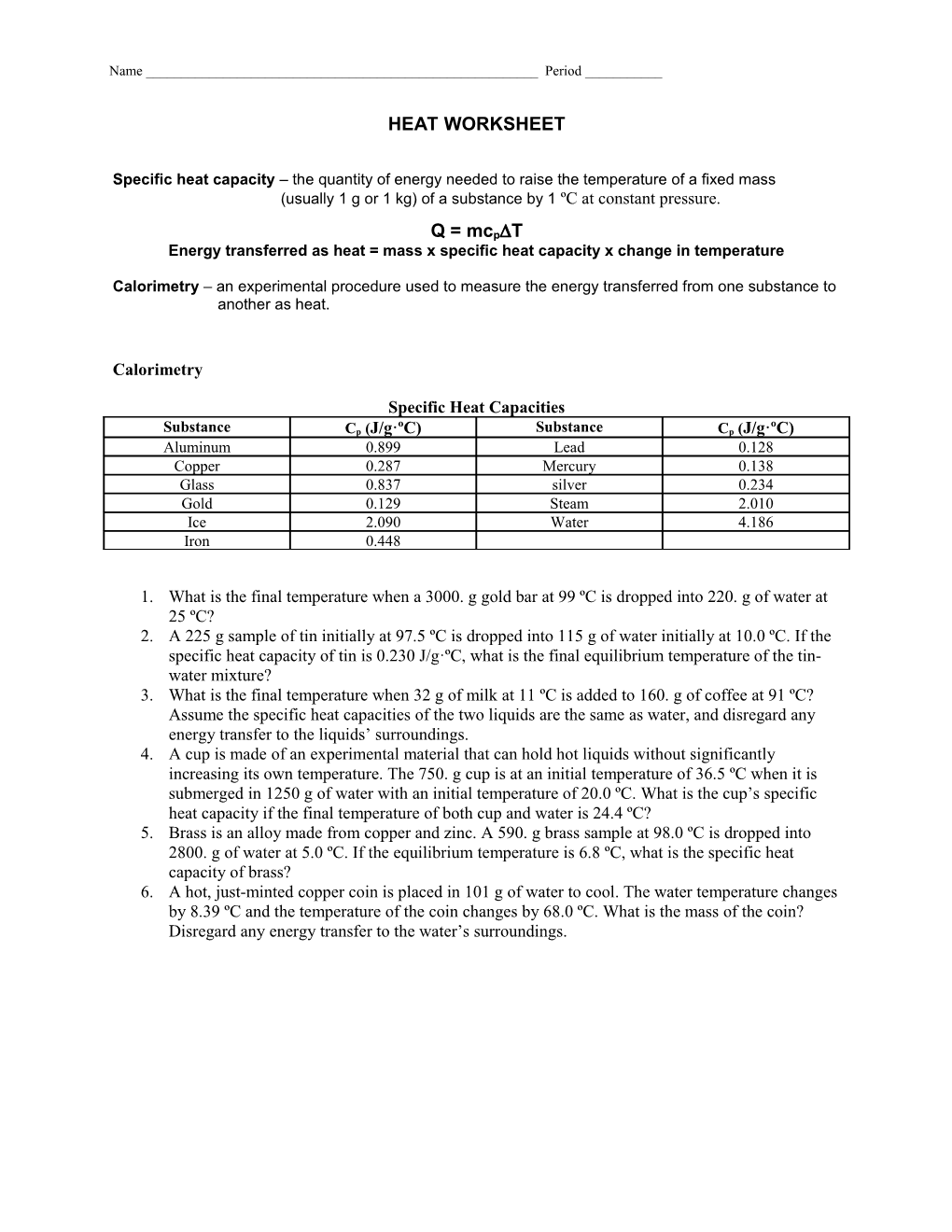# Energy Transferred As Heat = Mass X Specific Heat Capacity X Change in TemperatureHEAT WORKSHEET

Specific heat capacity – the quantity of energy needed to raise the temperature of a fixed mass

(usually 1 g or 1 kg) of a substance by 1 ºC at constant pressure.

Q = mcpT

#### Energy transferred as heat = mass x specific heat capacity x change in temperature

Calorimetry – an experimental procedure used to measure the energy transferred from one substance to another as heat.

## Calorimetry

##### Specific Heat Capacities
Substance / Cp (J/g·ºC) / Substance / Cp (J/g·ºC)
Aluminum / 0.899 / Lead / 0.128
Copper / 0.287 / Mercury / 0.138
Glass / 0.837 / silver / 0.234
Gold / 0.129 / Steam / 2.010
Ice / 2.090 / Water / 4.186
Iron / 0.448
1. What is the final temperature when a 3000. g gold bar at 99 ºC is dropped into 220. g of water at 25 ºC?
2. A 225 g sample of tin initially at 97.5 ºC is dropped into 115 g of water initially at 10.0 ºC. If the specific heat capacity of tin is 0.230 J/g·ºC, what is the final equilibrium temperature of the tin-water mixture?
3. What is the final temperature when 32 g of milk at 11 ºC is added to 160. g of coffee at 91 ºC? Assume the specific heat capacities of the two liquids are the same as water, and disregard any energy transfer to the liquids’ surroundings.
4. A cup is made of an experimental material that can hold hot liquids without significantly increasing its own temperature. The 750. g cup is at an initial temperature of 36.5 ºC when it is submerged in 1250 g of water with an initial temperature of 20.0 ºC. What is the cup’s specific heat capacity if the final temperature of both cup and water is 24.4 ºC?
5. Brass is an alloy made from copper and zinc. A 590. g brass sample at 98.0 ºC is dropped into 2800. g of water at 5.0 ºC. If the equilibrium temperature is 6.8 ºC, what is the specific heat capacity of brass?
6. A hot, just-minted copper coin is placed in 101 g of water to cool. The water temperature changes by 8.39 ºC and the temperature of the coin changes by 68.0 ºC. What is the mass of the coin? Disregard any energy transfer to the water’s surroundings.

Latent heat – the energy per unit mass that is transferred during a phase change of a substance.

Q = mL

### Heat of Phase Changes

##### Latent Heats of Fusion and Vaporization at Standard Pressure
Substance / Melting point (ºC) / Lf (J/kg) / Boiling point (ºC) / Lv (J/kg)
Nitrogen / -209.97 / 2.55 x 104 / -195.81 / 2.01 x 105
Oxygen / -218.79 / 1.38 x 104 / -182.97 / 2.13 x 105
Ethyl Alcohol / -114 / 1.04 x 105 / 78 / 8.54 x 105
Water / 0.00 / 3.33 x 105 / 100 / 2.26 x 106
Lead / 327.3 / 2.45 x 104 / 1745 / 8.70 x 105
Aluminum / 660.4 / 3.97 x 105 / 2467 / 1.14 x 107
1. How much energy is required to change a 42 g ice cube from ice at –11 ºC to steam at 111 ºC?
2. Liquid nitrogen, which has a boiling point of 77 K, is commonly used to cool substances to low temperatures. How much energy must be removed from 1.0 kg of gaseous nitrogen at 77 K for it to completely liquefy?
3. How much energy is needed to melt 0.225 kg of lead so that it can be used to make a lead sinker for fishing? The sample has an initial temperature of 27.3 ºC and is poured in the mold immediately after it has melted.
4. How much energy is needed to melt exactly 1000 aluminum cans, each with a mass of 14.0 g, for recycling? Assume an initial temperature of 26.4 ºC.
5. A .011 kg cube of ice at 0 ºC is added to 0.450 kg of soup at 80.0 ºC. Assuming that the soup has the same specific heat capacity as water, find the final temperature of the soup after the ice has melted. (Hint: There is a temperature change after the ice melts.)
6. At a foundry, 25 kg of molten aluminum with a temperature 660.4 ºC is poured into a mold. If this is carried out in a room containing 130 kg of air at 25 ºC, what is the temperature of the air after the aluminum is completely solidified? Assume that the specific heat capacity of is 1.0 x 103 J/kg·ºC.